## Multiply by one and ten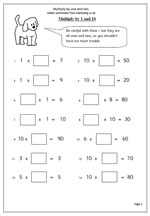We often presume that much of what young children have to do in maths is self evident and easily understood by them. However, often this is not the case, especially if children have missed some time or not had enough practice to consolidate their learning. Here we have a simple exercise to just check that children really know what happens when numbers are multiplied by one and ten.

This page can be found in our Year 2, Know Number Facts section.

Multiplying by 1 and 10

## Year 4 maths worksheet: multiples of 10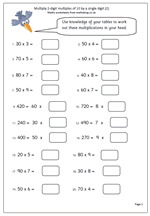The summer holidays are drawing to a close so what better for all those children just entering year 4 than a nice little mental arithmetic page. This page looks again at multiplying 2-digit multiples of 10 by a single digit. Dead easy if tables are known. If tables are not known this becomes much more difficult, so it is a good page to give to check that tables are sharp!

This page and many similar can be found in our Year 4 maths resources, Knowing Number Facts.

Multiply 2-digit multiples of 10_(p2)

## Maths worksheet: More multiplying decimals by 10 and 100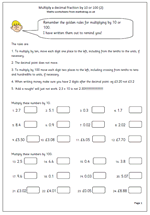Here we have another page on multiplying decimal fractions by 10 or 100. The method used today is to move each number one place to the left when multiplying by ten and two places to the left when multiplying by 100. The decimal point does not move. I seem to remember moving decimal points around when I was at school but this is certainly not recommended here.

When doing this with money it is worth remembering that two digits should always be written after the decimal point, so that £2.56 x 10 is not £25.6, but £25.60. (This is not an excuse for saying ‘add a nought’.)

Multiply a decimal by 10 and 100 (2)

## Maths worksheet: Multiply decimals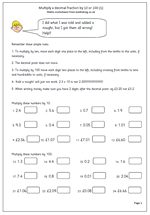There are some definite dos and don’ts when it comes to multiplying decimals ‘in your head’.

To multiply by 10 move each digit one place to the left, including tenths to the units, if necessary.

To multiply by 100 move each digit two places to the right.

The big don’t is to think that ‘add a nought’ is a short cut to multiplying by 10. 2.3 x 10 is not 2.30!!

This is a good check up page to make sure children understand what they are doing.

Multiply decimal fractions by 10 and 100 (1)

## Year 4 maths worksheet: Multiply multiples of 10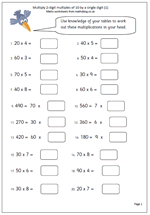This page is an excellent illustration of how knowing ‘tables’ and understanding place value can make other maths much simpler. These questions are all multiplying multiples of ten by a single digit.

If you know that 7 x 8 = 56 then 70 x 8 can be done ‘in your head’ very quickly.

probably the hardest questions on this sheet are those set out like this:

490 x 7 = ??

The best way to approach this is to ask what times 7 makes 49? and work from there.

Multiply 2-digit multiples of 10 (pg 1)

## Year 3 maths worksheet: Multiplying by 10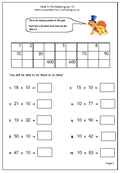We’re back on to the idea of multiplying a whole number by ten, probably one of the most important concepts in primary maths. Of course, by now we all know that each digit moves one place to the left and a zero is placed in the units, acting as a place holder for the other digits. It is easy to say ‘add a nought’ but this just doesn’t work later when decimals are multiplied by 10 and it doesn’t help to explain dividing by ten, when each digit moves one place to the right. Children can do simple multiplication by ten quickly, but they do need to understand what is happening.

Multiply by ten (p1)

## Multiply by 9 mentally (1)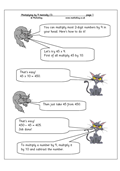You can multiply most 2-digit numbers by 9 in your head. The most efficient way is to multiply the number by ten and then subtract the number. For example:

45 x 9

First of all multiply 45 by 10 which is 450.

Then subtract 45 from 450 which is 405.

This maths worksheet shows how to do this and then gives some practice questions – but you do have to be pretty good at subtraction to use this method.

Multiply by 9 mentally (1)